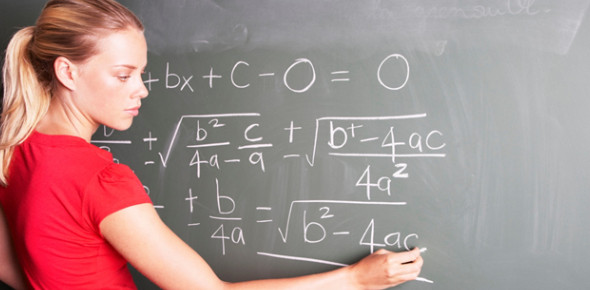Maths Quiz For Class 8

10 Questions | Total Attempts: 70194SettingsReady for an interesting Maths quiz for class 8? Math is all about having fun with numbers regularly. If you get to know that fact, you will never have any math problems. Do you believe that you can tackle these fun and easy questions below with ease? Give them a try and share your score in the comment section.

• 1.
How many feet are equal to a meter?
• A.

3.28 feet

• B.

2.28 feet

• C.

10 feet

• D.

20 feet

• 2.
If Hannah has 19.27\$ and Riley has 13.59\$, how much more money does Hannah have?
• A.

3.45\$

• B.

4.87\$

• C.

5.68\$

• D.

7.98\$

• 3.
How many right angles are there in a square?
• A.

1

• B.

2

• C.

3

• D.

4

• 4.
656 is a
• A.

Prime

• B.

Composite

• 5.
How many inches are equal to a foot?
• A.

10

• B.

11

• C.

12

• D.

14

• 6.
If you had 785 dogs, how many would you have if I took 524?
• A.

261

• B.

278

• C.

356

• D.

359

• 7.
Harmony had 357 cats, and Lindsey had 150 dogs, so how many animals are there altogether?
• A.

456

• B.

507

• C.

518

• D.

654

• 8.
Julian has 52 chocolates, and Bria has 169. How many are there altogether?
• A.

121

• B.

221

• C.

223

• D.

301

• 9.
If you want to go to Gurnee Mills, and it's 1 hour from your house, but you have to wait an extra hour, how many hours did it take you to get to Gurnee Mills?
• A.

2 hours

• B.

2:30 hours

• C.

3 hours

• D.

4 hours

• 10.
- 10 + 9 =
• A.

0

• B.

1

• C.

2

• D.

- 1

Related TopicsBack to top